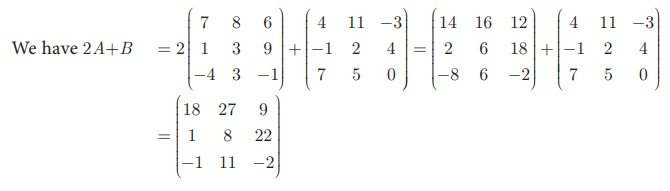Home | | Maths 10th Std | Addition and subtraction of matrices

# Addition and subtraction of matrices

In this section, we shall discuss the addition and subtraction of matrices, multiplication of a matrix by a scalar and multiplication of matrices.

Operations on Matrices

In this section, we shall discuss the addition and subtraction of matrices, multiplication of a matrix by a scalar and multiplication of matrices.

## Addition and subtraction of matrices

Two matrices can be added or subtracted if they have the same order. To add or subtract two matrices, simply add or subtract the corresponding elements.

For exampleIf A = (aij ) , B = (bij ) , i = 1, 2, ... m ,  j = 1, 2, ...then C = A + B is such that  C = (cij ) where cij = a ij +bij for all i = 1, 2, ... m and j = 1, 2, ... n

### Example 3.57### SolutionExample 3.58

Two examinations were conducted for three groups of students namely group 1, group 2, group 3 and their data on average of marks for the subjects Tamil, English, Science and Mathematics are given below in the form of matrices A and B. Find the total marks of both the examinations for all the three groups.Solution

The total marks in both the examinations for all the three groups is the sum of the given matrices.### Example 3.59### Solution

It is not possible to add A and B because they have different orders.

## Multiplication of Matrix by a Scalar

We can multiply the elements of the given matrix A by a non-zero number k to obtain a new matrix kA whose elements are multiplied by k. The matrix kA is called scalar multiplication of A.

Thus if A = (aij )m ×n  then , kA = (kaij )m ×n  for all i = 1,2,…,m and for all j = 1,2,…,n.

### Example 3.60

If A =then Find 2A+B.

### Solution

Since A and B have same order 3 ×3 , 2A + B is defined.Example 3.61Solution

Since A, B are of the same order 3 ×3 , subtraction of 4A and 3B is defined.Tags : Example Solved Problem , 10th Mathematics : UNIT 3 : Algebra
Study Material, Lecturing Notes, Assignment, Reference, Wiki description explanation, brief detail
10th Mathematics : UNIT 3 : Algebra : Addition and subtraction of matrices | Example Solved Problem